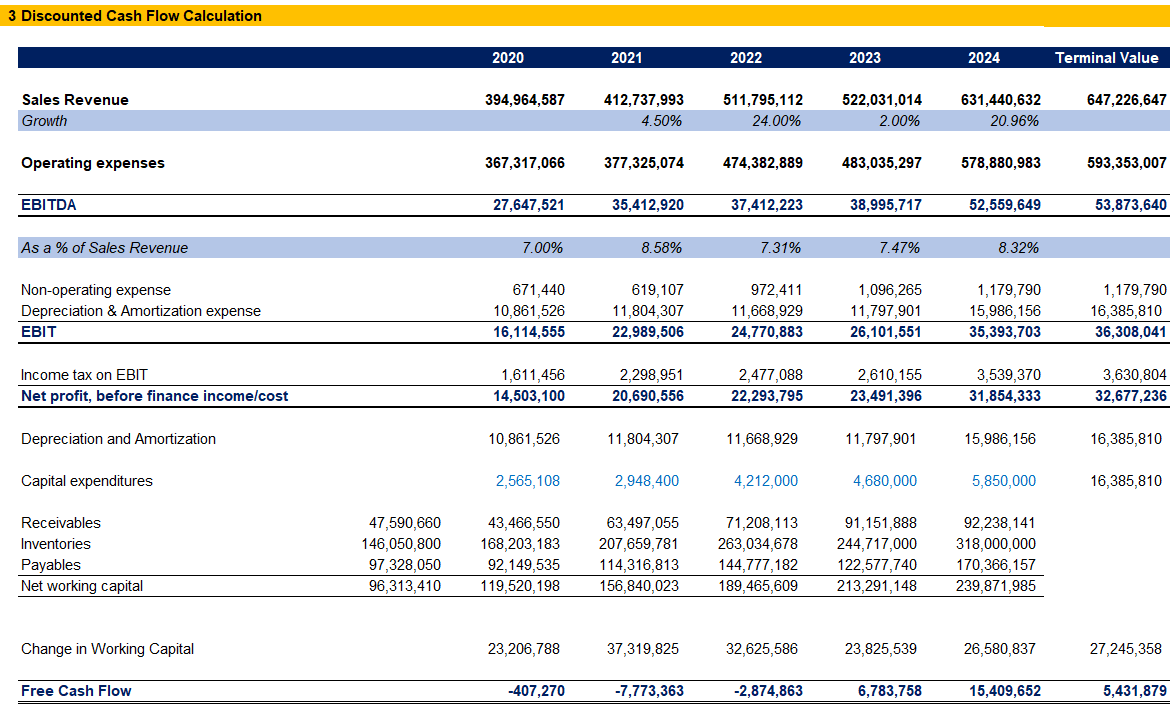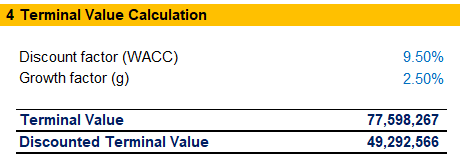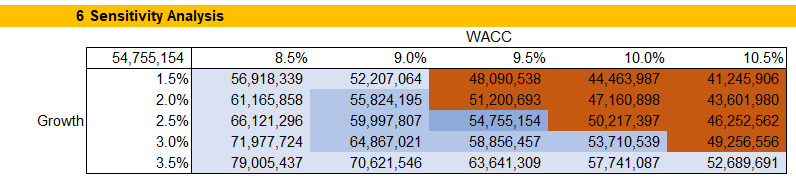## Calculate Value in Use under IAS 36

The core underlying principle of IAS 36 Impairment of Assets is that an asset’s carrying value in the financial statements of the company should not exceed the highest amount the business can recover through its use or sale.

The standard applies to all assets for which there are no impairment considerations elsewhere. As an example, when we test inventory for impairment, we follow IAS 2 Inventories, as it has specific stipulations concerning materials and goods.

For some assets listed in the standard, we calculate and evaluate the recoverable amount every year. These include goodwill on business combinations, assets with an indefinite useful life, and intangibles not yet ready for use. For all other assets (excluding such covered in other IFRS and IAS), we only perform the calculation when indications for impairment are present.

The following flowchart outlines the process under IAS 36.## Value In Use (VIU)

Under IAS 36, the recoverable amount is the higher of the asset’s fair value less cost to sell and its value in use. The latter is the present net worth of the asset or cash-generating unit (CGU).

This follows the assumption that there are two ways a company can get value out of an asset. We can either sell it, and the net proceeds will be the fair value less cost to sell, or we can use it and transfer benefits over to the business via the revenues or savings the asset generates less the associated costs.

To calculate the VIU, we estimate the present amount of the future cash flows that we expect to derive from the asset or CGU. Value in use represents the future expected cash flows from the continuing use of an asset and its disposal, discounted to reflect the underlying risk and the time value of money concept.

Impairment is then the difference between the carrying value and the recoverable amount.

## Calculation of Value in Use

We determine value in use for each separate asset. Whenever this approach is not practical, we calculate for the smallest identifiable CGU that contains the asset. We must be able to identify and separate the cash flows relevant to the CGU.

### Discount rate

Once we start with our value in use model, it is critical to determine the correct discount factor. The most proper discount rate is the one that the company can obtain to finance a separate asset with the same cash flows and risk patterns.

The discount factor should not be specific to the capital structure of the company. Therefore we use industry benchmarks by running comparative analysis against businesses operating in similar conditions.

In practice, the Weighted Average Cost of Capital (WACC) is the most common discount factor when we calculate the recoverable amount of an asset or CGU.

### Pre-tax and post-tax

As of now, IAS 36 requires that we calculate the value in use with pre-tax cash flows and a pre-tax discount rate. However, observable market rates are usually post-tax. In such cases, tax cash flows for VIU calculations are not the same as the company expects to pay. Ideally, we should calculate tax payments as if the tax base of the asset or CGU is equal to its recoverable amount. Such would be a very complex calculation, so we assume the tax base is identical to the carrying value of the asset. In other words, we use accounting depreciation to calculate the income tax charge for the value in use.

It’s essential to be consistent in determining the carrying value of a CGU and the related cash flows. For practical reasons, the VIU often includes items relating to working capital and others. Therefore, we have to remember to include these items in the carrying value as well.

Keep in mind that as the discount factor already covers the inherent business risks and the time value of money concept, we exclude interest and dividends from the value in use calculation.

## Example Value in Use Calculation

To shed more light on the VIU calculation, let us look at a practical model. We will prepare a Discounted Cash Flow (DCF) model for a cash-generating unit within a company.

First, we forecast the performance of the CGU for the next five years.To build the DCF model, we also need the changes in Working Capital over the period. Using our Income Statement forecast and our Cash Conversion Cycle, alongside some other assumptions, we can prepare a Balance Sheet forecast for the next five years.Now we can start building our DCF model. Remember, that we also include a column for our Terminal Value calculation. To calculate the Free Cash Flow elements in the terminal period, we use an expected growth of 2.50%. As we notice that Non-operating expenses increase less each year, we do not apply the assumption for growth on this line.

It is essential to remember that for capital expenditures in the final period, we do not apply the expected growth rate as well. Instead, we consider the terminal CAPEX to be equal to the Depreciation charge, as we expect those to be the same within the constraints of perpetuity.

With these specifics, we arrive at the company’s Free Cash Flow.Adding the WACC, we can now calculate the present value of the interim cash flows.

If you are interested in how to calculate the Weighted Average Cost of Capital (WACC) using the comparable companies approach, you can take a look at our case study, showing how to calculate WACC for Amazon.

Our Free Cash Flow also includes an additional column, which we use to calculate the Terminal Value of the CGU after the end of the forecast period. Remember that we need to apply the same discount factor as for the last year of our projections.The total of the Discounted Cash Flows and the Terminal Value of the CGU provides us with the indicated Value in Use.This is the value we use to compare to the CGU’s carrying value. In this case, the carrying amount is € 52 mil, so we have a headroom, and there is no need to recognize any impairment loss.

As discussed in our article on Sensitivity Analysis, in such cases, it is a great idea to add a sensitivity table showing how the value in use changes based on changes in the most critical assumptions for the expected growth rate and the WACC of the business.The Sensitivity table shows that the model is highly susceptible to changes in assumptions. If a single rate is off by 0.5%, the value in use will be below the carrying amount of the CGU. This indicates that we need to perform a critical assessment of our assumptions to make sure we are using the most proper values in our work.

## Conclusion

IAS 36 Impairment of Assets stipulates that the company has to present assets at the lower of their carrying value and recoverable amount. To do so, we often perform value in use calculations for specific assets, CGUs, or companies. We arrive at the expected future cash flows from the continuing use of the asset and its disposal, discounted to reflect the inherent risk and the time value of money. It is essential to be consistent when determining CGUs, their relevant cash flows, and the appropriate discount factor.

## And Get a FREE Benchmark Analysis Template## FCCA, FMVA

Hi! I am a finance professional with 10+ years of experience in audit, controlling, reporting, financial analysis and modeling. I am excited to delve deep into specifics of various industries, where I can identify the best solutions for clients I work with.

In my spare time, I am into skiing, hiking and running. I am also active on Instagram and YouTube, where I try different ways to express my creative side.

### 5 Responses

1. Marcela says:

Hello and thank you for the VIU analysis example. Can you please confirm that you are including the income tax expense and excluding the depreciation expense in your free cash flow calculation? Thank you.

1. Dobromir Dikov says:

Hi Marcela,
thank you for the question!
Yes, I am taking the after-tax result, you can read more on why in the part of the article called Pre-tax and post-tax.
As for the depreciation, I am starting from the post-depreciation result (EBIT), and then adding the depreciation back (eliminating the effects), as it is not a cash expense, and it doesn’t directly impact the cash flows.

2. Stephanie Kleovoulou says:

Hi, when calculating the VIU, is there a limit to the number of years of cash flows you can use in the DCF? Would it be limited to 5 years?

1. Dobromir Dikov says:

Hi Stephanie, there’s no requirement for how many years a DCF should include. The idea is to go as far as you can reasonably forecast the numbers. In most cases, I would try to forecast anywhere between 3 and 5 years before looking at the terminal value to cover the rest.

3. Mau says:

Thank you for these explanations. I have few questions:
1) In calculating changes in working capital is
(a) deferred tax asset/liability be considered in the computation?
(b) current income tax payable considered in the computation?
2) A company has only ordinary shares; it also has long term loans (this is not debenture) categorized as part of non-current liabilities. How is cost of capital be determined – is it going to be cost of equity alone (since it has only ordinary shares) or the cost of the long-term loan too will be considered? going to be WACC?
Thanks.